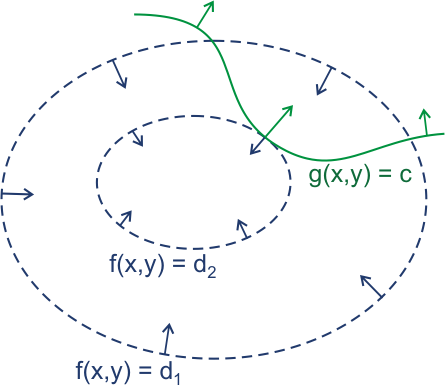0%

# Relation and Closure

$R$ is a relation on set $A$. If there exists a relation $S$ with property $P$ that includes $R$, and $S$ being a subset of all relations that has property $P$ and includes $R$, then $S$ is a closure of $R$ with respect to $P$.

## Reflexive Closure

for set $A$.

$\Delta=\{(a,a)|a\in A\}$.

For any $R$ on $A$, its reflexive closure is $R\cup\Delta$

## Symmetric Closure

for set $A$.

$R^{-1}=\{(b,a)|(a,b)\in R\}$.

For any $R$ on $A$, its symmetric closure is $R\cup R^{-1}$

## Transitive Closure

Connectivity Relation: $R$ is a relation on $A$. A connectivity relation $R^*=\bigcup_{n=1}^{\infty}R^n$.

Let $R’$ be the transitive closure of R, then $R’=R^*$.

Hence, to get the transitive closure of $R$, we need to calculate $M_{R^*}=\vee_{i=1}^nM_R^{[i]}$. $M_R$ is a 0-1 Matrix of $R$, which defined on n elements.

### Warshell Algorithm

Let $W_0=M_R$. $\forall k \in \{1,2,\cdots,n\}, W_k=[w_{ij}^{[k]}]$, $w_{ij}^{[k]}=w_{ij}^{[k-1]}\vee(w_{ik}^{[k-1]}\land w_{kj}^{[k-1]})$. $W_n=M_{R^*}$.

# Partial Order

$(S,\preccurlyeq)$ is a linear order(total order) set $\iff$ $\forall a,b\in S$,$a\preccurlyeq b$ or $b\preccurlyeq a$. A total order set is also called a chain.

For partial order set $(S,\preccurlyeq)$, if $\preccurlyeq$ is a linear order, and every non-empty subset of $S$ has a minimum element, we call it a well ordered set.

## Well-ordered Induction

$S$ is a well ordered set. $\forall y\in S$, if $P(x)$ is true for all $x\in S$ $\land$ $x\prec y$ leading to $P(y)$ is true, then $\forall x\in S, P(x)$ is true.

## Quasiorder

A relation $R$ on a set $A$ is called quasiorder if it is transitive and irreflexive.

## 数据格式

C声明 汇编后缀 大小(byte)
char b 1
short w 2
int l 4
long q 8
char* q 8
float s 4
double l 8

## 计算前缀函数

string substr (size_t pos = 0, size_t len = npos) const;

​​“看书只能落得孤独，对吧？”

“噢。”

“书那玩意是煮意大利面时用来打发时间才一只手拿着看的，明白？”

“嗯。”

“好——嘞……唔……看来我们可以交谈了。”

## 3:39

此时我所做的，正是一手拿着书，一手用筷子拨动着翻滚的沸水里的意大利细面条，等待它们逐渐由僵硬变软，进而富有弹性。宛如拯救一群冻僵的鲑鱼。

若是尚未由黑暗统治的白天，这种时候我习惯听Dry County。待Jovi第三次唱出we were just born to die的绝望之音时，锅中的面条软硬恰到好处，与加了橄榄的青酱正是绝配。但此刻是深夜三点，寂静之声正将自己推向高潮，响起这首长诗实在不合时宜。

对我而言，夜幕降临之后的顾影自怜，大多可以原谅。然而正如没有彻头彻尾的绝望，这世上没有完完全全的和解。在黑暗中自饮自酌，与自己长谈，到头来只能落得饥肠辘辘的下场。迫不得已，我一边捧着《路易·波拿巴的雾月十八日》，看马克思痛斥用拿破仑的死人铁面具掩盖自己丑陋面容的冒险家，一边任由锅中升起的氤氲湿气濡湿镜片。

厨房闷热如置身于盛夏的雨夜。

这便是唯一的出路。

## 拉格朗日乘数法的推导

求函数极值是微积分研究的重要问题。拉格朗日乘数法解决的核心问题就是对于自变量拥有约束条件（尤其是约束条件不能被化为显函数）的多元函数，如何求出其极值。以二元函数为例，对于函数$z=f(x,y)$,有约束条件$g(x,y)=c$.假设$z$在$(x_0,y_0)$处取得极值，并且$f(x,y),g(x,y)$在$(x_0,y_0)$的领域内都具有连续的一阶偏导数.

那么，首先借助几何直观来观察取得极值的必要条件.一直在回味这句话。

这正是对今天的世界的绝好概括。现今的世界正与黄金时代的开拓者们描绘的未来背道而驰：过去的47年间再没有仍何人登上月球，科技却围绕着信息技术极速发展，人类躺进了网络的温床。网络、虚拟现实的发展，神经科学对认知，对脑的研究……这些固然是正确的，但随之而来的产出（诸如网络的交互方式与刺激的升级），是否会使人类因为由此产生过于内向的视角而偏安一隅？

我仍然认同广袤的宇宙才是人类的边疆，是人类的想象力最好的去处和归宿。但如今人类的视角越发的集中在自我上，并通过沉浸入虚拟来对抗现实。正因此，黄金时代的壮丽太空不再是舞台的布景，取代它的是霓虹灯管，义肢，超级企业。

向信息世界的进军创造了无数价值，它无疑也是人类追寻美好的一种方式，但我仍然想念宇宙。

我痛恨没有星际航行的未来。只有信息技术的人类已经苍老，或许只有当人类迈着蹒跚的脚步，真正迈出这狭小无比的太阳系的时候，我们才风华正茂。正如刘所言：

我期待有那么一天，像那些曾经描写过信息时代的科幻小说一样，描写太空航行的科幻小说也变的平淡无奇了，那时的火星和小行星带都是乏味的地方，有无数的人在那里谋生；木星和它众多的卫星已成为旅游胜地，阻止人们去那里的唯一障碍就是昂贵的价格。

但即使在这个时候，宇宙仍是一个大得无法想象的存在，距我们最近的恒星仍然遥不可及。浩瀚的星空永远能够承载我们无穷的想象力。

在此不断感叹的我，却也走上了coding的道路。这也并非绝境。这就就不能将人类送向太空了吗？答案必然是否定的。

于是这也是愿望之一。

http://music.163.com/song?id=20866987&userid=58049281

ⅰ.不断重复的温馨的背景音乐早就停止了。此刻只剩下寂静之声在耳边轰鸣，响得可怕，仿佛要夺走我的一切感知。在阳光下，我尚且还能昂首向前，每当深夜造访，无助与孤寂便将我压到，使我动弹不得。

ⅱ.我恨自己生的太晚。我生存的年代，列侬早已死在粉丝的枪下，王小波死于心脏病。绿洲早已解散，小泽征尔垂垂老矣，Live Aid已经过去了34年。而录满爵士的LP大多消失的无影无踪——爵士通通变成了老歌，再也不是什么先锋音乐。

ⅲ.生活的一大主题就是寻找。无论你如何珍惜，有些东西注定要离去，消逝在风里。无可挽回。我们找寻故乡，想要寻得的却并非是那个处所。即使回到了物质的故乡，那里的空气与重力也早已改变。在这里，你仍然是悲哀的异乡人。我们怀恋故乡不只是那片土地。真正的故乡，是晚饭前的钟声，无数的欢喜伤悲，连同午后苦涩的阳光与寒冷彻骨的雪，我们寻它而不得，无非是因为故乡正是the nowhere land，哪里都不存在的地方，它是精神性的，那里永远生活着你曾经拥有，却注定离你而去的人。它是你不复返的孩提岁月，无可重复的青春。

ⅳ.我们是由现实铸造的。那么尚未被铸造的部分又是何物？

ⅴ.黑夜里，软弱是我的死敌。我又从何处寻得击败它的勇力？

Wish you were here.